# Square Planar Complexes - Coordination Chemistry Notes | Study Inorganic Chemistry - Chemistry

## Chemistry: Square Planar Complexes - Coordination Chemistry Notes | Study Inorganic Chemistry - Chemistry

The document Square Planar Complexes - Coordination Chemistry Notes | Study Inorganic Chemistry - Chemistry is a part of the Chemistry Course Inorganic Chemistry.
All you need of Chemistry at this link: Chemistry

Square Planar Complexes

In square planar complexes the central metal cation is either dsp 2 or sp2d hybridised. Examples are shown below:

[Ni(CN)4]2– ion:-In this complex the valence shell electronic configuration of Ni (o.s. +2) is 3d8. Since CN is a strong ligand, therefore, pairing of two unpaired electrons of 3d orbitals takes place resultin g in a vacant d orbital. This vacant 3d orbital gets hybridised with the vacant 4s and two of 4p orbitals to give four dsp2 hybrid orbitals. This lead to the formation of square planar geometry and the magnetic moment is zero. Hence the complex is diamagnetic.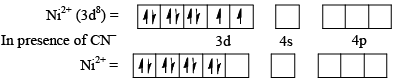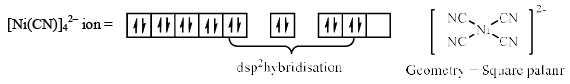n  = 0 ,       μ = 0 (diamagnetic)

[PdCl4]In this complex the o.s. of Pd is +2. Hence the outermost electronic configuration is 3d8. Alt hough Cl is a weak ligand, pairing of d electrons takes place because Pd is a transit ion metal of 4d series and according to VBT assumptions, the pairing always takes place in the 4d and 5d metals irrespective of the ligand type. Hence, 3d electrons get paired here. Consequently, dsp2 hybridisation takes place and the magnetic moment is zero. Thus, the geometry of this complex is square planar in contrast to corresponding Ni complex NiCl42– which is tetrahedral.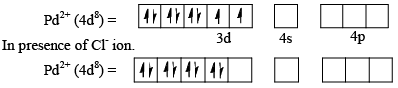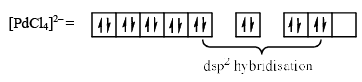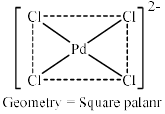n = 0,       μ = 0 (diamagnetic)

[Cu(NH3)4]2+In this complex ion, the o.s. of copper is +2 and its valence shell electronic configuration is 3d9. Magnetic moment observations indicate that the complex is paramagnetic. This observation is in correspondence with the tetrahedral geometry of this complex ion having sp3 hybridisation as shown below: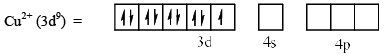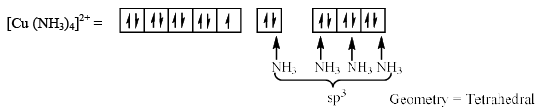n = 1,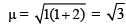= 1.73 B.M. (paramagnetic)

In this compound the expected hybridisation was sp3 but according to ESR (Electron Spin Resonance Spectroscop y) and X-ray crystallography studies, the structure of [Cu(NH3)4]2+ ion is square planar. But this is only possible with either dspor sp2d hybrid orbitals.
To form dsp2 hybrid orbitals, it is considered that the unpaired electrons in 3d orbital are promoted to the 4p orbital as shown below: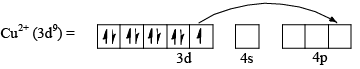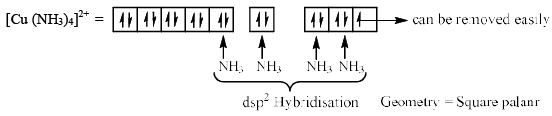In the above electronic configuration the single unpaired electron is present in the higher level 4p orbital. Hence it can be oxidised ver y easily. But the Cu 3+ ion does not exist i.e. oxidation of Cu2+ to Cu3+ is not possible.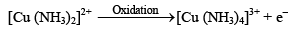This observation led to the failure of above theory of dsp2 hybridisation also.
Finally, it was suggested that in square planar [Cu(NH3)4]2+ complex, Cu2+ ion is sp2d hybridised as shown below.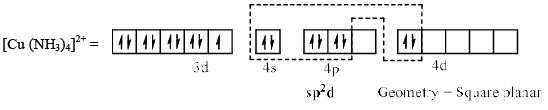In this configuration, one of the three 4p orbitals, 4pz orbital does not participate in hybridisation because pz orbital lies above and below the plane of the ion. [Cu(py)2]2+, [Cu(en)2]2+, [Cu(CN)4]2– all complexes are square planar and Cu2+ is sp2d hybridised.

Limitations of VBT

Although, VBT is useful to visualize the bonding in complexes but it fails to explain certain observations in coordination complexes:

• VBT does not explain the nature of ligands i.e. it does not tell which ligand is weak and which is strong.
• VBT could not explain the phenomenon of electron pairing which occurs in the presence of strong ligands.
• It does not explain the effect of temperature on magnetic moment and also not able to explain why the experimental value of magnetic moment is greater than the calculated value in some complexes.
• VBT could not explain the colour and electronic spectra of complexes.

Crystal Field Theory (CFT)

To explain the above properties which did not explain by VBT, Bethe and Van Vleck proposed another theory which is known as Crystal Field Theory or CFT.
This theory is based on following assumptions:

• Bonding in complexes is purely electrostatic in nat ure and not covalent as proposed b y the VBT.
• In complexes two types of electrostatic forces operate.
• One is the attraction between the metal cation and the e lectron rich ligands.
• The second type of electrostatic interaction is the repulsion between the lone pair of electrons on the ligands and electrons in the d orbitals of the metal cation and the repulsion between the two nuclei of metal cation and the ligand.
• Ionic ligands such as Cl, CN, OH are regarded as negative point charges or point charges and the neutral ligands such as H2O, NH3, py are regarded as point dipole. In the case of later species, the negative end of the dipole is directed towards the metal ion.
• The five orbitals in a free metal ion are degenerate. When a complex is formed, the electrostatic field of ligands affect the degeneracy of d orbitals and thereby destroy their degeneracy.
• The orbitals which lobes pointed towards axis of ligands have higher energies than those lying away from the ligands because of the repulsion between the d electrons and the ligands.

The shapes of five d orbitals are shown below: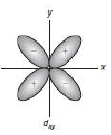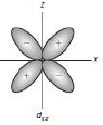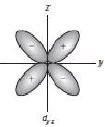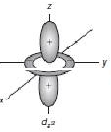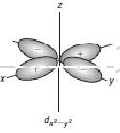All the three orbitals dxy, dyz and dzx lie in between the axes. These orbitals lie in xy, yz and zx-planes respectively. The dx2 – y2 orbital lie on x and y axes and the dz2 orbital on z-axis. All the five d orbitals are gerade because they have centre of symmetry exist between their axis. The (+) and (-) signs indicate the different phase of the lobes of orbitals.

The document Square Planar Complexes - Coordination Chemistry Notes | Study Inorganic Chemistry - Chemistry is a part of the Chemistry Course Inorganic Chemistry.
All you need of Chemistry at this link: ChemistryUse Code STAYHOME200 and get INR 200 additional OFF

## Inorganic Chemistry

49 videos|71 docs|16 tests

Track your progress, build streaks, highlight & save important lessons and more!

,

,

,

,

,

,

,

,

,

,

,

,

,

,

,

,

,

,

,

,

,

;